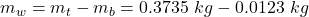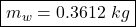## A bullet with a mass m b = 12.3 mb=12.3 g is fired into a block of wood at velocity v b = 249 m/s. vb=249 m/s. The block is attached to a sp

Question

A bullet with a mass m b = 12.3 mb=12.3 g is fired into a block of wood at velocity v b = 249 m/s. vb=249 m/s. The block is attached to a spring that has a spring constant k k of 205 N/m. 205 N/m. The block and bullet continue to move, compressing the spring by 35.0 cm 35.0 cm before the whole system momentarily comes to a stop. Assuming that the surface on which the block is resting is frictionless, determine the mass m w mw of the wooden block.

in progress 0
2 months 2021-07-28T01:06:18+00:00 1 Answers 5 views 0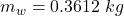Explanation:

Momentum and Energy

As the bullet-block system collide inelastically, the momentum is conserved before and after the collision. The energy is not conserved since the interaction is perfectly inelastic and part of the energy is lost  in the change of shape when both masses stick together.

Once the system moves on a frictionless surface, the kinetic energy is transformed into elastic energy of the spring.

The elastic energy of a spring of constant k being stretched by a distance x is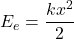The block+bullet system compresses the spring by x=0.35 m, and the spring’s constant is k=205 N/m, thus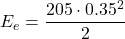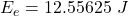The kinetic energy of both masses is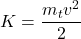Where mt is the total mass of the block (unknown) and the bullet. Equating this energy to the elastic energy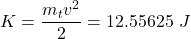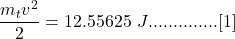Now consider the previous collision. The momentum before the collision is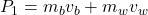where mb is the mass of the bullet, mw the mass of the block, and vb, vw their respective speeds. Since the block is assumed to be at rest: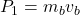The mass of the bullet is mb=12.3 gr=0.0123 Kg and its speed vb=249 m/s, thus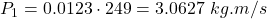The final momentum is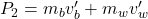Since both objects remain together, vb’=vw’=v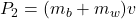Equating both momentums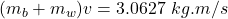The total mass mt is the sum of both masses, thus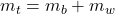Therefore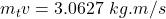Solving for v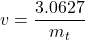Replacing in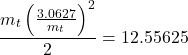Operating and simplifying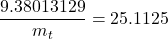Solving for mt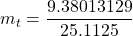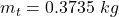Finally, the mass of the block alone is